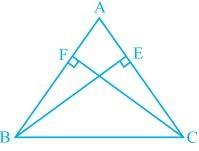# BE and CF are two equal altitudes of a triangle ABC. Using RHS congruence rule, prove that the triangle ABC is isosceles.#### Solution

Given

Given:

Altitude BE and CF are equal

To prove:

ΔABC is an isosceles Δ

In ΔBEC and ΔCEB

∠E=∠F —————-[each 90°]

BC=BC —————–[common]

BF=CF —————-[given]

ΔBEC ≅ ΔCEB [R.H.S]

∠C=∠B ————-[C.P.C.T]

In ΔABC,

∠C=∠B

Therefore, AB = AC as sides opposite to the equal angles is always equal.